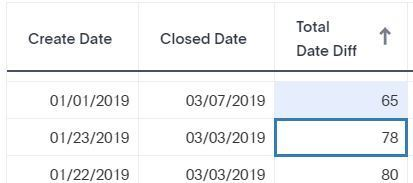# Formula: diff_daysHello, I am using diff_days formula to subtract one date from another; while some results are correct (1st row in attached image), some are not being calculated accurately (2nd row in attached mage). appreciate if someone can tell me what I am doing wrong.

Here is the formula I used:   diff_days ( closed date , created date )